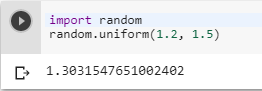2 views
in Python

randrange(start, stop) only takes integer arguments. So how would I get a random number between two float values?

by (106k points)

If you want to get a random number between a float range then you can use random.uniform(a,b), this function will return a random floating point number N where the range of N would be; a <= N <= b for a <= b and b <= N <= a for b < a.

import random

random.uniform(1.2, 1.5)